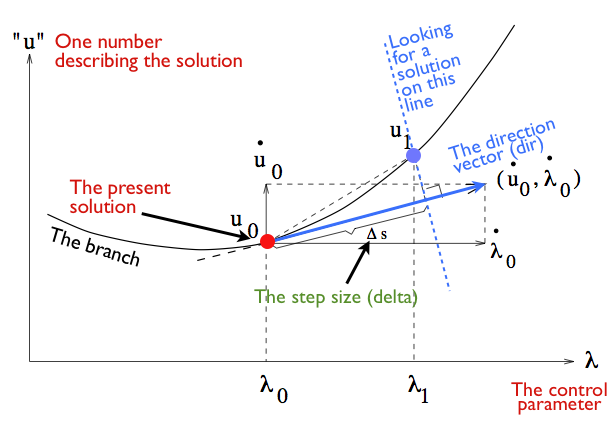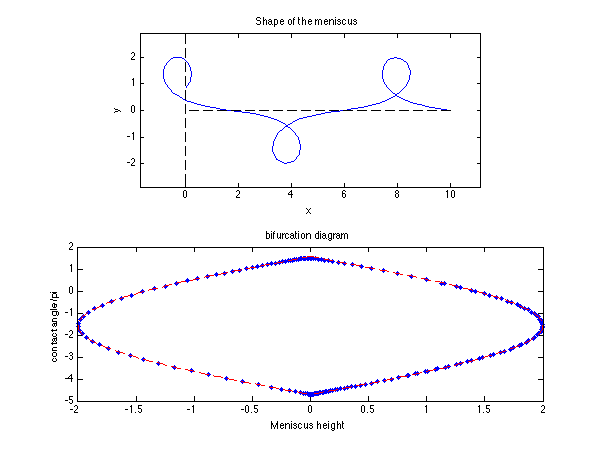# Keller’s arclength continuation on the meniscus

On previous examples we have done the computations of the shape of the fluid meniscus, and as done here, using a formulation that allows overturning of the interface. What we would like to do now is to do automatically the bifurcation diagram of this system, that is, vary the control parameter and see how the shape changes. For this we could simply do a loop and increase progressively our control parameter like for instance the contact angle at the wall or the meniscus height. But if you do this, you will have troubles with folds: places where you start to follow the nonlinear branch with increasing the control parameter and where you then need to decrease this parameter… This is the case for the bifurcation of the menisucs as you will see by running this code. Starting from the trivial flat solution, you can increase the height at the wall until a maximum value at which the contact angle is 0. Then the branch follows for negative contact angle and the meniscus height then decreases. A good place to learn about the technique of continuation is Eusebius Doedel’s lecture notes on the web site of his great code Auto.

The basic idea behind continuation is that a nonlinear solution cannot just disappear when varying a control parameter, it has to follow a branch. The is the persistence of nonlinear solutions. The second idea is that to follow a branch, it should not be you who decide the next value of the control parameter (the meniscus height for instance), but it should be the Newton step itself. So you leave the value of the control parameter as an unknown of your system, and you add one more equation that will define what should be the value of this parameter, depending on where you are on the branch, how big a jump you want to do at each step of the continuation and so on.

Basically, at one point of a branch, you need to have a direction vector: in which direction you want to look for a new solution on the branch, and a step size: how for on this direction do you want to look for the solution. Keller’s continuation method is a given choice on how to combine the direction and the distance, and it is sketched on the figure below.Keller’s continuation

clear all; clf;

% parameters
L=10;            % domain length
n=100;           % number of grid points
rho=1;           % density
sig=1;           % surface tension
g=1;             % gravity
delta=1;     % continuation length

% Differentiation matrices and integration
[D,DD,ws,s]=dif1D('cheb',0,1,n,3);
Z=zeros(n,n); I=eye(n);


# Initial guess

We now need to set up an initial guess at the same time for the shape of the meniscus, x, y, and \theta, but also for the value of the control parameter, which we have chosen here to be the eniscus height y(1). This value is the last element of the vector of unknowns. Then we start with a trivial choice of the initial direction, by saying that dir is a vector full of zeros, except of its last element. This way we say that we want the next solution to be in the direction of an increase of the meniscus height.

%initial guess
sol=[s;0*s;0*s; L; 0];
dir=[zeros(3*n+1,1);1];   % initial direction


# The continuation loop

We now have two loops: the outer loop is the continuation, it is basically moving along the nonlinear branch. Then we have an inner loop which cooresponds to the iterations of the Newton algorithm, to solve the nonlinear equations of the physical system (the shape of the meniscus) as well as the additional equation of how to choose the value of the control parameter: the height of the meniscus.

disp('Continuation loop')
ind=0;quitcon=0;
while ~quitcon&ind<200
solprev=sol;
sol=sol+dir*delta; % new prediction of solution

% Newton iterations
disp('Newton loop')
quit=0;count=0;
while ~quit

% the present solution and its derivatives
x=sol(1:n);
y=sol(n+1:2*n);
th=sol(2*n+1:3*n);
l=sol(3*n+1);
h0=sol(3*n+2);


# The nonlinear function and its jacobian

Here we compute the value of the nonlinear function f as we did before, except that there is now an additional equation dir'*(sol-solprev)-delta. This equation imposes that the scalar product of dirwith the vector that goes from the present solution to the new solution yet to be found, must be equal to delta, the step size.

Just after, we build the Jacobian cooresponding to this new nonlinear function. For the rows corresponding the physical system’s equations, we just add zeros where the height of the meniscus should come into play in the matrix-vector multiplication (here this parameter comes only in the boundary conditions). Then there is a last rwo that is the jacobian of the direction equation. Simply the jacobian is dir'. This Jacobian is easy because the condition is linear. Now one must be careful with one thing: the heigh of the meniscus comes into the fourth equation where we impose the value of y at the wall, thus there is a -1 in the column of the meniscus height.

       % nonlinear function
f=[ ...
l*cos(th)-D*x; ...
l*sin(th)-D*y; ...
rho*g/sig*l*y-D*th; ...
y(1)-h0; ...
dir'*(sol-solprev)-delta];

% analytical jacobian
A=[-D,   Z,  -l*diag(sin(th)),   cos(th),    Z(:,1); ...
Z,   -D, l*diag(cos(th)), sin(th),    Z(:,1); ...
Z,   rho*g/sig*l*I,  -D, rho*g/sig*y, Z(:,1); ...
Z(1,:),  I(1,:), Z(1,:), 0,  -1; ...
dir'];

% Boundary conditions
f([1 n+1 2*n+1])=[x(1)-0; x(n)-L; y(n)-0];
A([1 n+1 2*n+1],:)=[ ...
I(1,:),Z(1,:),Z(1,:),0,0; ...
I(n,:),Z(1,:),Z(1,:),0,0; ...
Z(n,:),I(n,:),Z(1,:),0,0];

% convergence test
res=norm(f);
disp([num2str(count) '  ' num2str(res)]);
if count>50|res>1e5; disp('no convergence');quitcon=1; break; end
if res<1e-5; quit=1; disp('converged'); continue; end


# Newton step

here is the solution of the linear system at each iteratino of the Newton interation. Nothing is changed, because this is just a Newton step. What has changed is the nonlinear functio that we solve and its Jacobian.

       % Newton step
sol=sol-A\f;
count=count+1;
end


# Showing the evolution

We display the evolutino of the computation during the computation to see if thigs are going well. On a first subplot we have the present shape of the meniscus, and in the second subplot below, we display the branch. For this we plot the contact angle at the wall versus the meniscus height. This is good because we can clearly see the fold.

   % ploting the present solution
if ~quitcon;
subplot(2,1,1);plot(x,y,'b-',[0,0],[-3,3],'k--',[0 L],[0 0],'k--');
xlabel('x'); ylabel('y');title('Shape of the meniscus')
axis([-2,L,-6,6]); axis equal;
subplot(2,1,2); plot(y(1),th(1)+pi/2,'b.');
xlabel('Meniscus height'); ylabel('contact angle/pi'); title('bifurcation diagram');
drawnow;hold on
end

% New direction
dir=A\[zeros(3*n+1,1);1]; % new direction
dir=dir/norm(dir); % normalization

ind=ind+1;
end


# Comparison with theory

Now we do as we should always do: compare this result with something else. Here we continue with the solution from the force balance that we have used for the other examples of the meniscus. We show this expression between the height of the meniscus and the contact angle at the wall as a red line. Here note that the contact angle is called \beta and is the angle bewtween the wall and the interface, which is different from the variable \theta, which is the angle between the horizontal and the interface.

% add the theory for comparison
bvec=linspace(-3*pi/2,pi/2,100);
plot(sqrt(2*(1-sin(bvec))),bvec,'r-',-sqrt(2*(1-sin(bvec))),bvec,'r-');


# Results

Here is the figure. Here we show the shape of the solution after many steps, where the interface has allready done a self-contact, so this is nolonger a physical solution. But it is nice to see the robustness of the algorithm, and the bifurcation diagram with two folds. You can as well see that there is a nice fit between the computation and the nonlinear theory (in red).Keller’s continuation of the overturning meniscus